Mobile QR Code1. (Department of Game Software, Hongik University / Sejong, Korea jang1na2@gmail.com, {hykim, bseo}@hongik.ac.kr)
2. (Department of Computer Science, Oklahoma State University / Stillwater, OK, USA npark@cs.okstate.edu )

Blockchain, Digital signature, ECDSA, Ethereum, Schnorr

## 1. Introduction

As the interest in Bitcoin  increases worldwide, there are more opportunities for researching and developing blockchain. Bitcoin was initially shunned by people to the extent that it was called bubbles and fraud'', but now it is developing by combining various technologies. Since Vitalik Buterin published the Ethereum  blockchain platform, technology research, and applicable functions have progressed rapidly in the blockchain industry. On the other hand, ECDSA, a digital signature adopted by Ethereum, which is currently being used in the blockchain digital signature system, has a problem with efficiency.

A Schnorr digital signature method was proposed to solve these problems and study why Schnorr should be used by comparison with ECDSA. Section 2 describes the elliptic curve and elliptic curve cryptography, which are the basic backgrounds of the blockchain digital signatures. Section 3 outlines the digital signature algorithms for the ECDSA and Schnorr digital signatures. Section 4 explains how the Schnorr digital signatures are more efficient than ECDSA by showing the test environment and the results for comparative testing of ECDSA and Schnorr. Schnorr has more strengths than ECDSA in the gas price, block size, signature size, block elapsed time, and signature algorithm calculation time. Hence, digital signatures that apply the Schnorr were implemented in a cryptographic approach using a private Ethereum network .

## 2. Background and Related Work

### 2.1 Elliptic Curve

The cryptographic background of the blockchain digital signature is elliptic curve cryptography. Elliptic curve cryptography is produced based on the Discrete Logarithm Problem (DLP) and the algebraic structure of elliptic curves on finite-body fields. The equation form of the elliptic curve is expressed as Eq. (1), and the expression of this equation is called the Weierstrass equation form.

##### (1)
$y^{2}=x^{3}+ax+b,\,\,\,\,\left(a,b\in K\right)$

The Standards for Efficient Cryptography (SEC), founded by Certicom in Canada, provide standardized information for the widespread application of elliptic curve cryptography. These elliptic curves require parameters that must be selected carefully because the elliptic curve used is determined by these parameters. There are various ways to select a parameter. For example, it may be generated randomly. Non-random curves are explicitly formed when selecting verifiable random parameters. Fig. 1 provides an example of an elliptic curve .

##### Fig. 1. Examples of the Elliptic curve.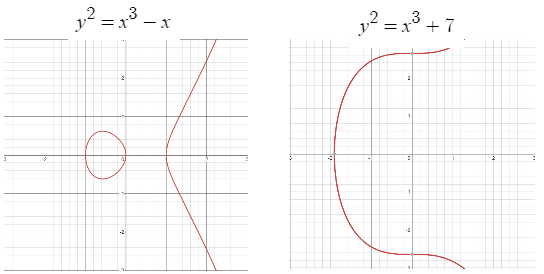##### (2)
$T=\left(p,a,b,G,n,h\right)$

There are various standardized elliptic curves that include these characteristics to ensure efficient computation. T in Eq. (2) is a tuple that expresses the six parameters required for the elliptic curve by the expression. p denotes the boundary of the finite field of the elliptic curve. The a and b are coefficients that determine the shape of the finite field elliptic curve in Eq. (1). G is the constructor point that exists above the elliptic curve. n is the value generated by circulating the subgroup of G. h is a cofactor required for signature .

### 2.2 Elliptic Curve Cryptography (ECC)

#### 2.2.1 Hasse’s Theorem

Fig. 2 shows the typical shape of the elliptic curve for the finite field; it is no longer a smooth curve but a set of points, as shown in Fig. 1. A finite series of points is scattered along the plane. It is symmetric on the x-axis of $\frac{p}{2}$ and can define a geometrical method for point addition. The general idea is to draw a line that passes like the considered point $Q_{1}$ and $Q_{2}$. This line is tracked along itself through modular calculation. Once the line crosses the point $-Q_{3}$, the opposite point $Q_{3}$ is selected to have the result of addition. On the other hand, this approach can be confusing because it is depicted in pictures. Therefore, it is expressed in algebraic notation. $E\left(F_{p}\right)$ is defined as E (Elliptic Curve), F (Finite Field), and p (Prime Number). The number of points on the elliptic curve relative to the $E\left(F_{p}\right)$ is determined by p. This is called Hasse’s theorem or the boundary of Hasse .

##### Fig. 2. $y^{2}=x^{3}-x+3\left(\mathrm{mod127}\right)$ Hasse’s theorem.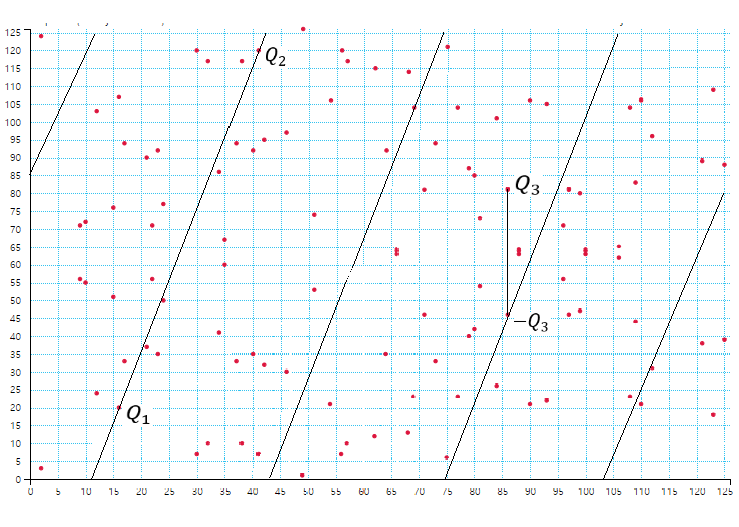#### 2.2.2 Lagrange’s Theorem

According to Lagrange’s theorem, if $E\left(F_{p}\right)$ is a prime number, then $E\left(F_{p}\right)$ is a cyclic group. Lagrange’s theorem is that the size of the subgroup of the finite group is a subgroup of the original size of the group. The order of each subgroup is the group order. Hence, it is a subgroup when the group order is a prime number. This means that the entire group of G must exist. If G is fixed, it should be added repeatedly. It will then reach a different point in the $E\left(F_{p}\right)$. Eventually, it reaches infinity. We would have found a cyclic subgroup when the private key is equal to the order of G. Therefore, if $E\left(F_{p}\right)$ is a prime number, the entire set of points in the elliptic curve forms a cyclic group .

#### 2.2.3 Discrete Logarithm Problem (DLP)

DLP is a matter of determining the k integers in $x^{k}=y$. In other words, it is to find a $k=log_{x}\,.$ Security cryptography systems based on ECC are determined by the difficulty of the DLP defined through the Elliptic Curve Discrete Logarithm Problem (ECDLP). This becomes difficult and impractical when k is an integer with hundreds of bits, which is a huge number because SECP256K1 is 256 bits. Furthermore, the time complexity to find the DLP increases to $O\left(2^{256}\right)$. The main advantage of ECC is that the encryption key size is small. The DLP difficulty is still difficult; the security level is the same, and the storage and transmission requirements can be reduced. In the execution time of the elliptic curve algorithm, elliptic scalar operations require a certain amount of time in milliseconds. ECDLP will require a period of $10^{28}$ years if calculated approximately. Even with today's supercomputers, the calculations are computationally impracticable. For this reason, DLP is the core of many public key cryptosystems .

#### 2.2.4 SECP256K1

The elliptic curve currently used by Ethereum for digital signatures is a curve called SECP256K1. To explain the definition, SEC is the Standards for Efficient Cryptography (SEC). P is the parameter representing the size of the boundary of the finite field. P comes after 256 bits, which represents the length in bits. The field size suggests that solving the DLP in the elliptic curve is difficult. K stands for the Koblitz curve and is distinct from the random curve, representing verifiable random use. Finally, it is sequence number 1, which is divided for discriminating the elliptic curves. SECP256K1 is composed of a special method and has the equation, $y^{2}=x^{3}+7,$ to ensure efficiency. The parameters for SECP256K1 configuration are defined, as listed in Table 1 .

##### Table 1. Parameters for ECDSA (secp256k1).
 Parameter Value p FFFFFFFF FFFFFFFF FFFFFFFF FFFFFFFF FFFFFFFF FFFFFFFF FFFFFFFF FFFFFC2F a 0 b 7 G 79BE667E F9DCBBAC 55A06295 CE870B07 029BFCDB 2DCE28D9 59F2815B 16F81798, 483ADA77 26A3C465 5DA4FBFC 0E1108A8 FD17B448 A6855419 9C47D08F FB10D4B8 n FFFFFFFF FFFFFFFF FFFFFFFF FFFFFFFE BAAEDCE6 AF48A03B BFD25E8C D0364141 h 01
##### Table 2. Experiment Parameters.
 Description Parameter Private key value q Message value m Random coefficient value k Message leftmost bits z ECDSA signature coefficient v, r, s Schnorr signature coefficient s, e Public key value Q Generator point G SHA256, keccak256 hash function hash ABI Encoded to 32bytes bytes32 Modular calculation mod

## 3. Algorithms used in the Experiment

### 3.1 Elliptic Curve Digital Signature Algorithm (ECDSA)

ECDSA is an elliptic curve-based digital signature algorithm used in Ethereum. It was proposed as an alternative to the RSA encryption method used in the past. It is an elliptic curve encryption technology that performs faster with small numbers of encryption keys than the longer encryption keys with slower encryption speeds. ECDSA is very difficult to infer a private key based on ECDLP encryption technology. That is, knowing the generator point G and the public key does not mean that the private key is known. On the other hand, ECDSA currently has various problems in efficiency, and the cases are as follows.

· High gas price, Large signature size, block size

· Inverse modular calculation, which means many inverse modular calculations are required.

· Scalar multiplication, in which the highest calculations are required.

These problems are a very slow task, which is valid for everything only if n is a prime number; n must be a prime number because of the difficulty of the ECDLP. The process involves taking the x-coordinate of the function K used in step 5 and modular n (Fig. 3). This means that the attacker cannot find r, but the random value k cannot ignore the probability of satisfying the equation in step 5 $r=x_{K}\left(\mathrm{mod}n\right)$ [10,11].

The following explains how the ECDSA signing algorithm was implemented. Fig. 3 shows that it has a message and q (private key) as the input values. k is generated as a random value, and z is the leftmost bit number of messages hashed with the keccak256 function. If r zero in the modular calculation in step 5, then return to step 3 and generate the k random value again. The s signature value was made by inputting $s=k^{-1}\left(z+rq\right)\left(\mathrm{mod}n\right)$ into the equation. If the s zero, return to step 3 and generate the k random value again. Finally, return values are public keys, s, and r signatures. How the ECDSA verification algorithm was implemented will be described. Fig. 4 shows that it has a message, public keys, s, and r signature values as the input values. z denotes the leftmost bit number of the same message, as shown in Fig. 3. To make the kG of step 6, the equations $u_{1},$ $u_{2}$ are defined and $kG=~ u_{1}G+u_{2}Q$. Finally, the r signature received as an input is compared with K, which has performed a modular calculation. The verification was performed as Eqs. (3)-(6) below [10,11].

##### Fig. 4. ECDSA verification algorithm.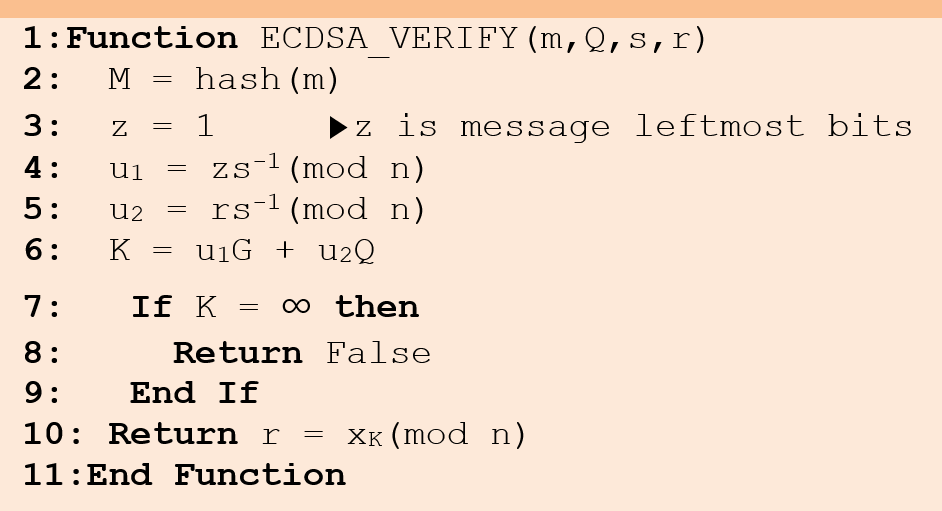##### (3)
$u_{1}G+u_{2}Q=zs^{-1}G+rs^{-1}Q=~ s^{-1}\left(z+rq\right)G \\$
##### (4)
$=\left(k^{-1}\left(z+rq\right)\right)^{-1}\left(z+rq\right)G \\$
##### (5)
$=k\left(z+rq\right)^{-1}\left(z+rq\right)G \\$
##### (6)
$=kG=K$

Furthermore, there is a way to accelerate the verification process; it is Straus’s algorithm, the sum of the two scalar multiplications. It is also known as Shamir’s trick, which can be calculated faster than the two separate scalar multiplications in the sixth step in Fig. 4. When K is made efficiently, the result can be obtained in less time, in which case Fig. 4 multiplied by s in the sixth step becomes the $sK=zG+rQ$ equation, so inverse modular calculations are not required. This can be achieved by including the K point [10,11].

### 3.2 Schnorr Digital Signature

Following Peter Wuille’s recent Bitcoin Improvement Proposal (BIP), Schnorr’s standardization of Bitcoin protocols is explained. As specified in BIP, ECDSA is standardized, whereas Schnorr signatures are not because they have had patent problems over the past few years. On the other hand, Schnorr itself is better in many ways than ECDSA, and it tries proving and analyzing the results through the test. Schnorr uses fewer signatures than ECDSA and there is no inverse modular calculation. The efficiency is better in terms of the speed of function calculation. Schnorr can be deployed easily through a soft fork. The Schnorr signature can enable easier implementation of some of the protocols required. Because Schnorr is not standardized, design selection is possible. Schnorr usually uses the P-256 curve released by NIST in the United States, and uses the sec256r1 curve by the SEC. The parameters for constructing the Barreto-Naehrig (BN) curve for the Schnorr digital signature were defined, as shown in Table 3 [11-13].

This section explains how the Schnorr signing algorithm was implemented. Fig. 5 shows that it has a message and q (private key) as input values. In the k value, the private key and the message are hashed with the keccak256 hash function, and two public keys (x, y) coordinates are obtained by multiplying the generator point G in q by the elliptic curve scalar. Find the equation $e=hash(kG|Q|M)$ required for Schnorr signature and make $s=k-eq$ the equation. The public keys, s, and e signature values are outputs as return values. The following describes how the Schnorr verification algorithm was implemented. Fig. 6 has a message, Q (public keys), s, and e as input values resulting from the signature algorithm. If the public key has an infinite value or does not exist in the elliptic curve, the return value is False. As long as the equation $kG=sG+eQ$ is performed, the result of hash with the e signature value received as an input value is compared with the equation $e=hash(kG|Q|M)$. If it is wrong, return False, else if right return True. Eqs. (7)-(9) performed the verification below. ECDSA has an inverse function, but Schnorr does not [11,13].

##### (7)
$sG+eQ=\left(k-eq\right)G+eqG \\$
##### (8)
$=kG-eQ+eQ \\$
##### (9)
$=kG=K$
##### Fig. 5. Schnorr signing algorithm.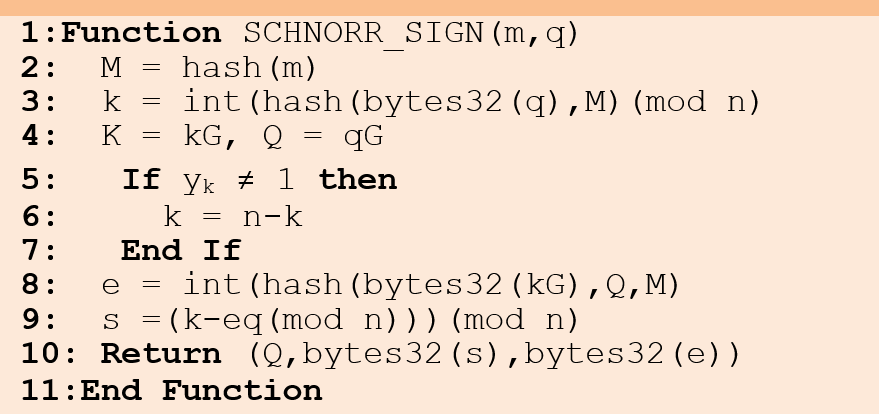##### Fig. 6. Schnorr verification algorithm.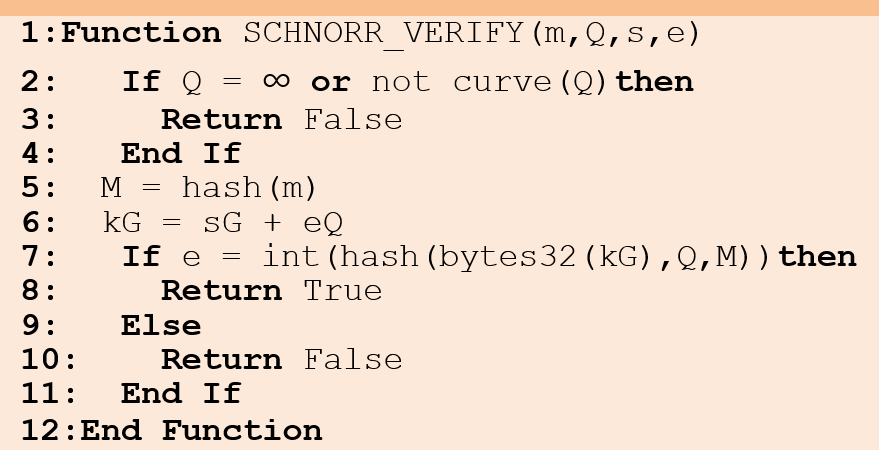##### Table 3. Parameters for Schnorr (BN Curve).
 Parameter Value p 0x30644e72e131a029b85045b68181585d9 7816a916871ca8d3c208c16d87cfd47 a 0 b 3 G 115597320329863871079910040213922857839 2581286182119253091740315145239 1805634, 408236787586343368133220340314543556831 685132759340120810574107621412 0093531 n 0x30644e72e131a029b85045b68181585d2 833e84879b9709143e1f593f0000001 h 01

## 4. Performance Analysis

Table 4 lists the test environment. Ethereum is the most representative blockchain platform developed by Vitalik Buterin based on Bitcoin in July 2015. Ethereum can access the main network, test network, and private network through the Go-Ethereum (Geth) client. Geth [2,3] is a client that allows access to the Ethereum blockchain network, and it is written in the Go language. The so-called Ganache, which corresponds to the private network, was used.

Solidity language [2,3] was designed by the Ethereum Solidity team, starting with Gavin Wood’s proposal in August 2014, to implement the Ethereum smart contract functionality operating on EVM in a static type of programming language. The Solidity language is compiled into byte codes that can operate on EVMs. Developers can implement the applications by putting their business logic into smart contracts using Solidity language. Items recorded in smart contracts cannot be denied and cannot be modified once deployed, so they must be written carefully.

Remix IDE  is an integrated development environment site that enables integrated coding, compilation, debugging, and deployment of Solidity languages on a browser. In other words, it is a site where Solidity codes can be written and compiled on the Internet. It can be used in a web browser without a separate installation, has its own compiler ‘solc’, and can access Ethereum's network. Furthermore, it includes a private Ethereum network, such as Ganache, which was used in the present study.

The above figures show the process of proving the Schnorr digital signature implemented in Remix IDE. The CreateProof function is an algorithm that signs a Schnorr digital signature (Fig. 7). Secret means a private key, and message can be viewed as message values to be signed. It can be seen that two public key values, s and e signature values appear as result values as output values. In Fig. 8, the VerifyProof function is an algorithm that verifies s, e signatures, public keys, and messages as input values in the Schnorr signature algorithm. As shown in Fig. 8, because the message value is signed 0x1111, entering a different message value of 0x1112 results in False. In Fig. 9, it is confirmed that the signature message value must match 0x1111 to become True. The Schnorr signature algorithm was confirmed to be working correctly; it was deployed in the present study for testing in the private Ethereum network environment.

##### Table 4. Test Environment.
 Component Value Geth 1.10.1 Version with Go language 1.16 Version Solidity Solidity 0. 8. 7 Version Complier CPU Intel Core i3-8100 3.60Ghz (4 CPUs) SSD KingDian N580 256 GB RAM 8 GB Operating System Windows 10 Application Remix IDE, Web3 HTML
##### Fig. 7. Proof of the Schnorr signature.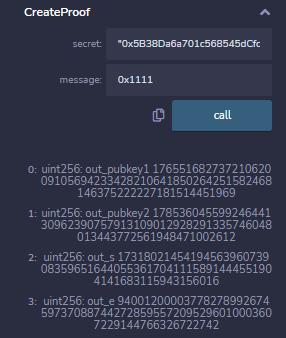##### Fig. 8. Proof of Schnorr verification (false).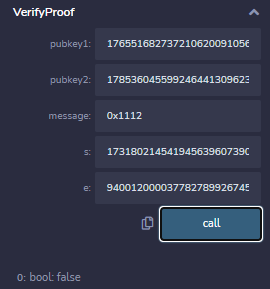##### Fig. 9. Proof of Schnorr verification (true).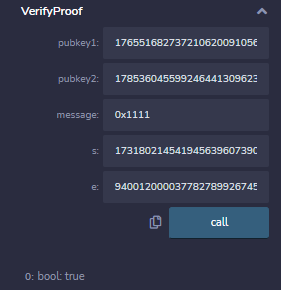Figs. 10 and 11 show that the next step was to confirm whether the Schnorr and ECDSA contracts were well deployed in the private Ethereum network. In Fig. 12, it can be confirmed that Schnorr and ECDSA are successfully recorded in the blockchain network. Schnorr’s gas price is 664052, and ECDSA’s gas price is 865141. Schnorr was recorded in the 212th block. The number of transactions (txs) value rose by 1. The block mining and sealing times were 11.968ms and 153.617ms, respectively, totaling 165.585ms. The ECDSA contract was recorded in the 385th block. Similarly, the number of transactions (txs) value increased by 1. The block mining and sealing elapsed times were 18.823ms and 392.950ms, respectively. The total block elapsed time was 411.773ms. Figs. 13 and 14 show that the block size was 3022bytes in the 212th block, and the block size was 3784bytes in the 385th block. Both Schnorr and ECDSA contracts have the same miner as 0xf03a27b9de2abf495e7c9b1a0fbd80b4f74908d2.

##### Fig. 10. Schnorr contract deployed.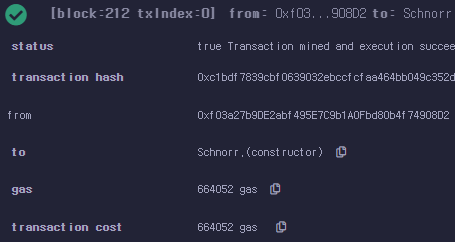##### Fig. 11. ECDSA contract deployed.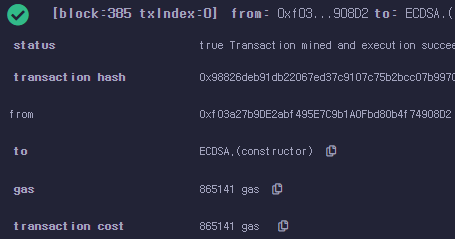##### Fig. 12. Schnorr, ECDSA recorded in 212th, 385th blocks.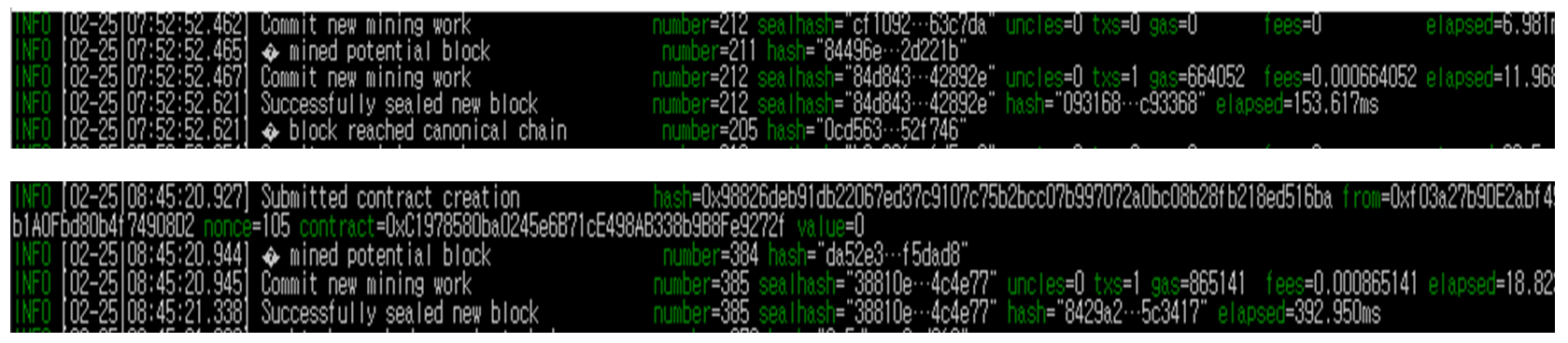It is recommended to save this contract hash value separately. This is because several smartcontracts will be placed if the blockchain is used, which could cause problems in using them because they could lose hash values later. The deployed smartcontracts were stored in the Ethereum blockchain, and their attributes were examined. Moreover, approximately 600 blocks were mined for the simulation, and 100 experiments were conducted for the Schnorr and ECDSA. The process of the simulation is shown in Fig. 12.

##### Fig. 13. Schnorr 212th block component.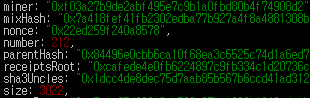##### Fig. 14. ECDSA 385th block component.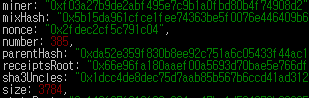ECDSA uses 70-72bytes (DER encoding) for the signature, and Schnorr uses fixed-sized 64 bytes. More specifically, ECDSA has v (6-8bytes), r (32bytes), and s (32bytes) signatures. Schnorr has s (32bytes) and e (32bytes) signatures. Because ECDSA generally includes a recovery parameter to support public key recovery, and Schnorr has a y-coordinate as a parameter in one x-coordinate in the signature, the public key recovery becomes possible without a recovery parameter. Moreover, regardless of function, the gas price is determined by size. Hence, size is a critical issue when producing Ethereum smart contracts. In Fig. 15, the gas price, Schnorr was 664052, and ECDSA was 865141. Schnorr could reduce the gas price by approximately 23.24% compared to ECDSA. Figs. 16 and 17 show that the Schnorr signature size is 11.11% smaller than ECDSA, and Schnorr block size is 20.13% less than ECDSA. Owing to the characteristics of most blockchain, the blocks continue to be produced, and the size continues to grow in proportion to time. Therefore, Schnorr can reduce this growth of blockchain size and has good efficiency than ECDSA .

Fig. 18 shows that elapsed time in both ECDSA and Schnorr. The experiments were deployed 100 times, and obtain the results with a graph. The Schnorr and ECDSA average times were 445.07ms and 596.97ms, respectively. Schnorr was 25.44% faster than ECSDA. On the other hand, in the case of a private Ethereum network, there are some variations every time the expanded time works, so it needs to be taken into the Ethereum Main network. The signature algorithm calculations are point multiplication, scalar multiplication, and inverse operation. In Eqs. (10) and (11), reference  pointed out that the one scalar multiplication operation satisfies the requirement of 923 multiplications. The inverse operation was approximately equal to 10 multiplication operations. In Table 5, ECDSA has four point multiplications (Point Mul.), two inverse operations (Inverse), and three scalar multiplications (Scalar Mul.). Schnorr has four point multiplications, zero inverse operation, and two scalar multiplications. ECDSA used 2803 multiplication calculation times, and Schnorr used 1850 multiplication calculation times. The signature calculation time of Schnorr was 34% faster than that of ECDSA. In other words, ECDSA has heavier operations, such as inverse operations and scalar multiplications in the calculation process, while Schnorr was relatively small, so the Schnorr signature calculations operate faster than ECDSA [16,17].

##### Fig. 15. Schnorr, ECDSA gas price.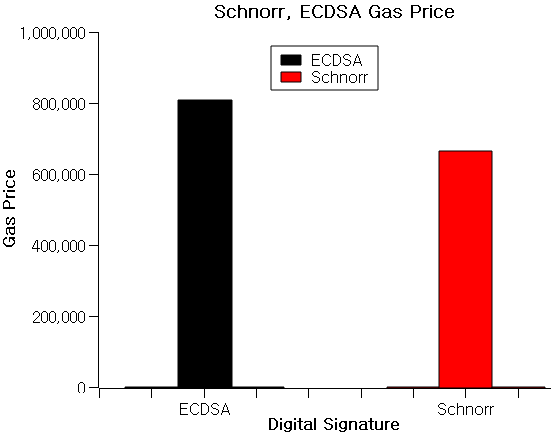##### Fig. 16. Schnorr, ECDSA signature size.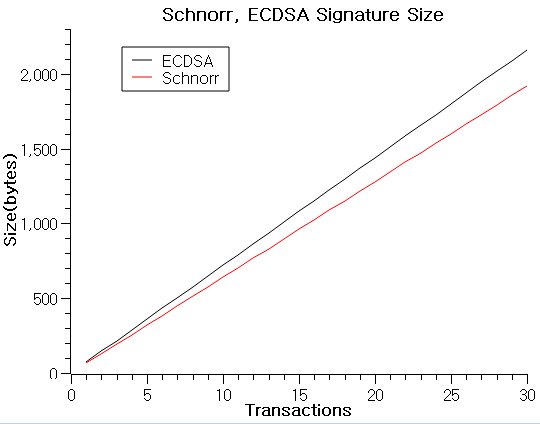##### Fig. 17. Schnorr, ECDSA block size.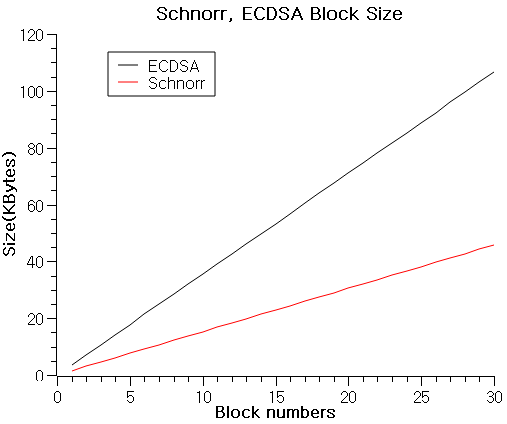##### Fig. 18. ECDSA, Schnorr block elapsed time.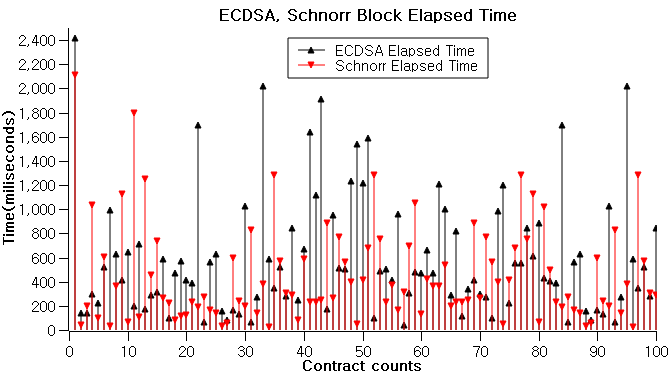##### Table 5. ECDSA, Schnorr amount of calculation and Block elapsed time[16,17]
 Digital signature Point Mul. Inverse Scalar Mul. Total Block elasped time (Average) Sign Verify Sign Verify Sign Verify ECDSA 2 2 1 2 1 2 2803 mul 596.97ms Schnorr 2 2 0 0 1 1 1850 mul 445.07ms
##### (10)
$1Inverse=10Mul$
##### (11)
$1Scalar\,\,Mul=923Mul$

## 5. Conclusion

This study implemented and tested a digital signature using Schnorr instead of ECDSA currently used in Ethereum. The two plans were compared while studying the problems of ECDSA and solved using Schnorr. Schnorr has strengths in efficiency. The efficiency is approximately the size and speed of both algorithms. More specifically, it is the gas price, signature size, block size, block elapsed time, and algorithm calculation time. Schnorr has superior efficiency to ECDSA. On the other hand, these results were conducted on a private Ethereum network; it may be different on a public Ethereum network. The last thing to highlight was that the Schnorr advantages presented in this study were not all the advantages Schnorr has. Further research will be needed on the technical aspect and the possibilities of Schnorr in the blockchain.

### ACKNOWLEDGMENTS

This study was supported by the National Research Foundation of Korea (NRF) grant funded by the Korean government (MSIT) (No.2019R1A2C1008533), and this work was supported by the 2022 Hongik University Research Fund.

### REFERENCES

1
Nakamoto S., 2008, Bitcoin: A peer-to-peer electronic cash system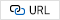2
Buterin V., 2014, Ethereum white paper, Ethereum3
Antonopoulos A. M., Wood G., 2018, Mastering Ethereum: Building smart contracts and dapps, O’Reilly Media, Inc.4
Brown D. R. L., May 2009, SEC 1: Elliptic Curve Cryptography, Standards for Efficient Cryptography, Certicom Research5
Brown D. R. L., 2010, SEC 2: Recommended Elliptic Curve Domain Parameters, Standards for Efficient Cryptography, Certicom Research6
Corbellini A., 2015, Elliptic Curve Cryptography: finite fields and discrete logarithms [Internet]7
,Lagrange’s Theorem(group theory) [Internet], Wikipedia8
Chen L., Moody D., Regenscheid A., Randall K., 2019, Recommendations for Discrete Logarithm-Based Cryptography (NIST 800-186 Draft), National Institute of standards and technology (NIST)9
2019, Digital Signature Standard (FIPS 186-5 Draft), National Institute of standards and technology (NIST)10
Bi W., Jia X., Zheng M., 2018, A Secure Multiple Elliptic Curves Digital Signature Algorithm for Blockchain, arXiv preprint arXiv:1808.0298811
Soldati G., 2018, An Advanced Signature Scheme: Schnorr Algorithm and its Benefits to the Bitcoin Ecosystem, Master’s thesis, Politecnico di Milano, Italy12
Barreto P. S. L. M., Naehrig M., 2006, Pairing-Friendly Elliptic Curves of Prime Order, Selected Areas in Cryptography-SAC 2005. volume 3897 of Lecture Notes in Computer Science, pp. 319-33113
Alegro J. K. P., Arboleda E. R., Perena M. R., Dellosa R. M., Oct. 2019., Hybrid Schnorr, RSA, and AES Cryptosystem, International Journal of Scientific & Technology Research, Vol. 8, No. 1015
Khalique A., Singh K., Sood S., 2010, Implementation of Elliptic Curve Digital Signature Algorithm, International Journal of Computer Applications (0975-8887), Vol. 2, No. 216
Liu S. G., Chen W. Q., Liu J. L., 2021, An Efficient Double Parameter Elliptic Curve Digital Signature Algorithm for Blockchain, IEEE Access17
Hong-Mei W. U., 2010, Elgamal digital signature scheme based on the elliptic curves, Journal of ChuXiong Normal Univ., Vol. 25, No. 3, pp. 44-47## Author

Jangho Na received his A.S. degree in Game development from Chunnam Techno University, South Korea, in 2018. He received his B.S. degree in Game Software from Hongik University, South Korea, in 2021. Since 2021, he has been a Master’s student at the School of Games at Hongik University, Sejong, South Korea. His research interests include blockchain, Ethereum, digital signature, NFT, Game servers, and cryptography.

Hye-Young Kim received her Ph.D. in Computer Science and Engineering from Korea University, Seoul, South Korea, in February 2005. During her Ph.D. study, she focused on location management schemes and traffic modeling, such as Mobile IPv6, cellular network, and mobility. In 2006, she was a Post-Doc. at the Wright State University (WSU). She has worked at Hongik University of South Korea as a full professor since March 2007. She developed a network protocol for nine years while she was working at Hyundai Electronics as a senior researcher. Her research interests include traffic modeling and load balancing scheme applying deep learning in IoT and Blockchain.

Nohpill Park received his B.S and M.S degrees in Computer Science from Seoul National University, Seoul, Korea, in 1987 and 1989, respectively, and Ph.D. degree from the Texas A&M University, College Station, in 1997. He is currently an Associate Professor at the department of computer science, Oklahoma State University, Stillwater. His current research interests include computer architecture, defect and fault-tolerant systems, testing and quality assurance of digital systems, parallel and distributed computer systems, multichip module systems, and programmable digital systems.

Beomjoo Seo received the B.S. and M.S. degrees from the Department of Computer Engineering, Seoul National University, Seoul, Korea, in 1994 and 1996, respectively, and the Ph.D. degree in computer science from the University of Southern California, Los Angeles, CA, USA, in 2008. His research at the University of Southern California was supervised by Dr. Roger Zimmermann. He worked for 5 years as a Research Engineer at LG Electronics, Seoul, Korea. He was formerly a Research Fellow at the School of Computing, National University of Singapore, Singapore. He is currently an Assistant Professor at the School of Games, Hongik University, Sejong, Korea. His research includes distributed storage model, distributed spatial audio streaming for virtual worlds, and sensor-rich mobile video acquisition, annotation, and its application.